Kenneth Levasseur
Mathematical Sciences
UMass Lowell
Kenneth_Levasseur@uml.edu

Calculations done here use AbstractAlgebra a Mathematica package available at http://www.central.edu/eaam/index.asp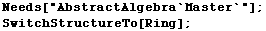You add and  multiply polynomials over an arbitrary ring the same way you learned to do it for polynomials over the real number in high school algebra.  The only thing you have to keep in mind is that the coefficient operations use the underlying operations for the ring over which you are working.  For example,  if you working with polynomials over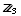then adding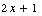and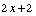involved adding "like terms" and the result isGiven two polynomials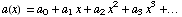Their sum is the polynomialYou just add corresponding coefficients to add.   Notice that we didn't specify exactly where either two of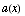and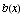"stop" but eventually the coefficients of both are zero and the number of additions in the underlying ring is no more than the largest degree of the two addends.

Example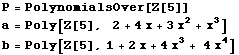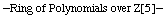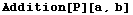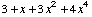Multiplication

Given two polynomials of degree m and n, respectively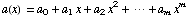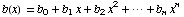Their product is the polynomials is computed by multiplying all of the terms in the first polynomial by each term in the second.  This involves multiplying powers of the indeterminate x, which follows the rule, where the addition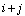is of integers, not in the underlying ring.   If we organize our results the product follows a regular pattern, but somewhat more complicated than for addition.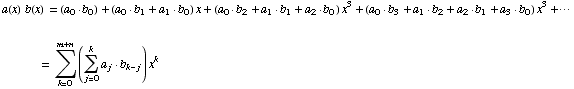In both of the formulae above it should be understood that coefficients with index higher than the respective degrees are equal to the zero of the underlying ring.    Notice that for each term in the product the coefficient ofis the sum of products of coefficients whose indices add up to k.

In multiplying polynomials of degree m and n, the number of multiplications involving nonzero coefficients can be as high as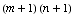Example

This product isn't as easy to see as the sum above.  Work out a few coefficients to check the result.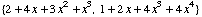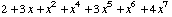Here's why we will usually work with polynomials over a field.  The following strange things don't happen if a ring doesn't have zero divisors as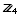has.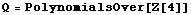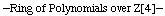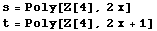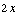A zero divisor among the polynomials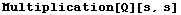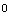Here we see that t is a unit, which isn't bad; but normally we expect the degree of a product to be the sum of degrees, but not in this polynomial ring.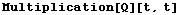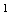Over a field this strange result never occurs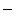the product of two linear polynomials is always a quadratic polynomial.

Created by Mathematica  (September 13, 2005)